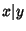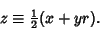## Lagrange Number (Diophantine Equation)

Given a Fermat Difference Equation (a quadratic Diophantine Equation)witha Quadratic Surd, assign to each solutionthe Lagrange numberThe product and quotient of two Lagrange numbers are also Lagrange numbers. Furthermore, every Lagrange number is a Power of the smallest Lagrange number with an integral exponent.